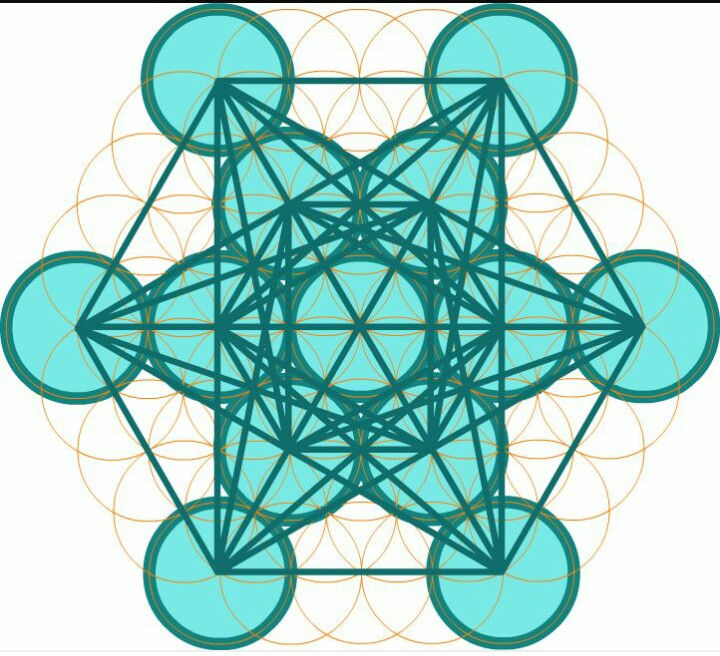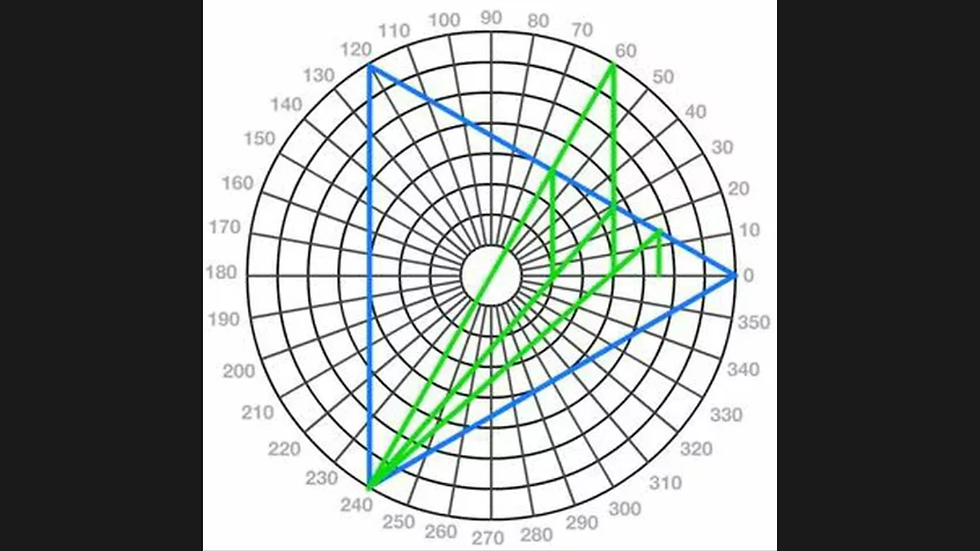top of page
Search

# 64 tetrahedron grid fruit of life part 3

(Fruit of life)=(metatrons cube)=((64 tetrahedron grid(E8(2 tetragrammatons(tetractys))))=(8 tree of life's(E8xE8)))=(8 enneagram(8 star tetrahedrons))

Tetragrammatons=72=7:2=7:14=7:77

7:77=777=161616=444=4³=(64 tetrahedron grid)

(Tetragrammaton)=(tetractys)=(10dimensions)=(star tetrahedron)=(tree of life)=(seed of life)=(fingerprint of god)=(enneagram)=(triangle numbers):Tetractys=2³+2³+2²=dodecahedron=(star tetrahedron)=33

33=33:33=66=enneagram